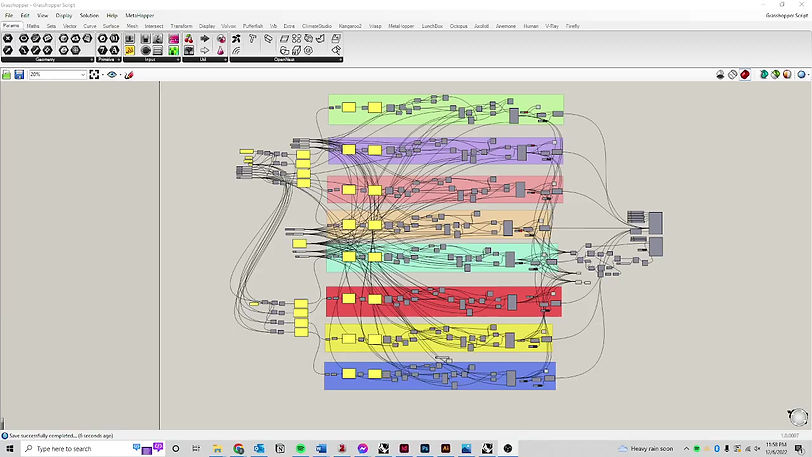top of page

# FIGURE TAXONOMY

an alphabet of ballroom dance steps

The notation of movements of the body has been a topic actively explored since the 17th century. The project explores the opportunities in representing figures of smooth ballroom dance styles - the waltz, the tango, and the foxtrot, in three dimensions.

## CONTEXT

Drawing ++

MIT Media Lab

p5js, Open Frameworks, Grasshopper, Rhinoceros3D, Photoshop, Premiere, Illustrator, 3D Printing

Zach Liberman

## SOFTWARE

#### DANCE NOTATION

##### a history

In the 1680s Pierre Beauchamp developed a dance notation, the Beauchamp-Feuillet notation, which was used to record Baroque dance styles.

The Smooth dance styles explored in this project are similarly a progressive dances, which means they are directionally moving dances made up of specific collections of steps.

#### DEVELOPING A 3D NOTATION

##### a processvideo isolation of leader and follower

in order to track both, only one can be tracked at a time

let _USE_LIVE_VIDEO = false;

let landmarks;

let W = 640;
let H = 360;

// https://mediapipe.dev/images/mobile/pose_tracking_full_body_landmarks.png
let connects = [0,15,13,11,12,14,16,23,24,25,26,27,28];

function onResults(results) {
if (results.poseLandmarks && results.poseLandmarks.length){
landmarks = results.poseLandmarks;
}
}

const pose = new Pose({locateFile: (file) => {
return `https://cdn.jsdelivr.net/npm/@mediapipe/pose/\${file}`;
}});
pose.setOptions({
modelComplexity: 1,
smoothLandmarks: true,
enableSegmentation: true,
smoothSegmentation: true,
minDetectionConfidence: 0.5,
minTrackingConfidence: 0.5
});
pose.onResults(onResults);

let capture;

async function send(){
if (capture.elt.width){
await pose.send({image: capture.elt});
}
setTimeout(send,10);
}

let sel;
let tex=[];
let color = []
let tex_w = 10;

function draw3D() {
noFill();
for (let i =0; i< limbs.length; i++){
Line[i].push([landmarks[limbs[i]].x*W,landmarks[limbs[i]].y*H,landmarks[limbs[i]].z*H]);

texture(tex[i]);
makeMesh(Line[i]);
}
}

function setup() {
pixelDensity(1);
createCanvas(W,H,WEBGL);

tex = createGraphics(tex_w,tex_w);
tex.stroke("green");
tex.line(0,tex_w,tex_w,tex_w);

tex = createGraphics(tex_w,tex_w);
tex.stroke("red");
tex.line(0,tex_w,tex_w,tex_w);

tex = createGraphics(tex_w,tex_w);
tex.stroke("yellow");
tex.line(0,tex_w,tex_w,tex_w);

tex = createGraphics(tex_w,tex_w);
tex.stroke("blue");
tex.line(0,tex_w,tex_w,tex_w);

if (_USE_LIVE_VIDEO){
capture = createCapture({video:{mandatory:{maxWidth:W,maxHeight:H}},audio:false});
}
else {
capture.play();
}
capture.hide();

setTimeout(send,10);

}

let trace = [];
let pos = [];
let USE_ORTHO = false;
let limbs = [15,16, 31, 32];
let Line = new Array(limbs.length).fill(0);
for (let i=0; i<limbs.length; ++i) Line[i] = [];

function draw() {
background(220);
translate(-W/2,-H/2);

image(capture,0,0,W,H);

if (landmarks){
//     fill(255,255,0);
//     noStroke();
//     landmarks.forEach(({x,y})=>circle(x*W,y*H,2));

stroke(255);
strokeWeight(2);
noFill();

draw3D();
}
}

function clamp(a,b,c){
return min(max(a,b),c);
}

function getSmoothed(poly,smoothingSize,smoothingShape=0){
// https://github.com/openframeworks/openFrameworks/blob/c21aba181f5180a8f4c2e0bcbde541a643abecec/libs/openFrameworks/graphics/ofPolyline.inl#L470
let n = poly.length;
smoothingSize = clamp(smoothingSize, 0, n);
smoothingShape = clamp(smoothingShape, 0, 1);

// precompute weights and normalization
let weights = new Array(smoothingSize);
// side weights
for(let i = 1; i < smoothingSize; i++) {
let curWeight = map(i, 0, smoothingSize, 1, smoothingShape);
weights[i] = curWeight;
}
// make a copy of this polyline
let result = poly.map(xy=>[...xy]);
let bClosed = false;
for(let i = 0; i < n; i++) {
let sum = 1; // center weight
for(let j = 1; j < smoothingSize; j++) {
let curx = 0;
let cury = 0;
let leftPosition = i - j;
let rightPosition = i + j;
if(leftPosition < 0 && bClosed) {
leftPosition += n;
}
if(leftPosition >= 0) {
curx += poly[leftPosition];
cury += poly[leftPosition];
sum += weights[j];
}
if(rightPosition >= n && bClosed) {
rightPosition -= n;
}
if(rightPosition < n) {
curx += poly[rightPosition];
cury += poly[rightPosition];
sum += weights[j];
}
result[i] += curx * weights[j];
result[i] += cury * weights[j];
}
result[i] /= sum;
result[i] /= sum;
}
return result;
}

function keyPressed() {
if (keyCode === ENTER){
for (let i =0; i< limbs.length; i++){
Linev = lineV(Line[i]);
}
}
}

function lineV (LineI)
{
let Linev = [];
for (let i = 1; i < LineI.length; i++){
let p0 = LineI[i-1];
let p1 = LineI[i];
let d = Math.hypot(p0-p1,p0-p1,p0-p1);
Linev.push([...p0,d]);
}
return Linev;
}

var element = document.createElement('a');
element.setAttribute('href', 'data:text/plain;charset=utf-8,' + encodeURIComponent(text));
element.style.display = 'none';
document.body.appendChild(element);
element.click();
document.body.removeChild(element);
}

function getResampledBySpacing(poly0,spacing) {
if(spacing==0 || poly0.length == 0) return poly0;
let poly = [];
let acc_len = ;
let tot_len = 0;
for (let i = 0; i < poly0.length-1; i++){
let [x0,y0] = poly0[i];
let [x1,y1] = poly0[i+1];
tot_len += Math.hypot(x1-x0,y1-y0);
acc_len.push(tot_len);
}
function getPointAtLength(l){
for (let i = poly0.length-1; i >= 0; i--){
if (acc_len[i] <= l){
let t = (l - acc_len[i])/(acc_len[i+1] - acc_len[i]);
return [
poly0[i] * (1-t) + poly0[i+1] * t,
poly0[i] * (1-t) + poly0[i+1] * t,
];
}
}
return [0,0];
}

for (let f = 0; f < tot_len; f += spacing){
poly.push(getPointAtLength(f));
}
if(poly.length) poly.push(poly0[poly0.length-1]);
return poly;
}videos are run through the code

after the video is played, four spreadsheets, one for each limb, are exportedspreadsheets input into grasshopper script to generate geometry

the spreadsheets are parsed and a curve is drawn from the points in the first three columns, the speeds in the fourth column are used as the radii for the pipegeometry backed into rhino

the rhino geometry can then be rendered or exported for 3D printing

#### THE WALTZ

##### an elegant, progressive dancewaltz mashup

waltz figure alphabet

underarm turn, side by side, reverse turn, hesitation with turn, grapevine, right box turnwaltz 3D model

#### THE TANGO

##### a sharp, progressive dancetango mashup

tango figure alphabet

tango walk, rock step, medis corto, left rock step, la peurta, flare promenadetango figure turntables

tango walk, la peurta, flare promenadetango 3D model

#### THE FOXTROT

##### a smooth, progressive dance